Courses

# Chemistry Test 12 - Mole Concept, Atomic Structure, Periodic Properties, Nomenclature, Isomerism

## 30 Questions MCQ Test Mock Test Series for JEE Main & Advanced 2022 | Chemistry Test 12 - Mole Concept, Atomic Structure, Periodic Properties, Nomenclature, Isomerism

Description
This mock test of Chemistry Test 12 - Mole Concept, Atomic Structure, Periodic Properties, Nomenclature, Isomerism for JEE helps you for every JEE entrance exam. This contains 30 Multiple Choice Questions for JEE Chemistry Test 12 - Mole Concept, Atomic Structure, Periodic Properties, Nomenclature, Isomerism (mcq) to study with solutions a complete question bank. The solved questions answers in this Chemistry Test 12 - Mole Concept, Atomic Structure, Periodic Properties, Nomenclature, Isomerism quiz give you a good mix of easy questions and tough questions. JEE students definitely take this Chemistry Test 12 - Mole Concept, Atomic Structure, Periodic Properties, Nomenclature, Isomerism exercise for a better result in the exam. You can find other Chemistry Test 12 - Mole Concept, Atomic Structure, Periodic Properties, Nomenclature, Isomerism extra questions, long questions & short questions for JEE on EduRev as well by searching above.
QUESTION: 1

### The density of chlorine relative to air is

Solution: Clhas a molecular mass of 71 g. And molecular mass of Air is 29 g .
Relative density of chlorine =( Mass of chlorine/ Volume of chlorine) / (Mass of air/ Volume of air )
Volume of any gas at STP = 22.4 l Relative density of chlorine = (71 / 22.4 ) / (29/22.4)
Relative density of chlorine = 2.44
QUESTION: 2

Solution:
QUESTION: 3

### How many moles of electrons wieght one kilogram ?

Solution:
QUESTION: 4
10 mL of gaseous hydrocarbon on combustion gives 40 mL of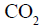(g) and 50 mL of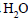(vap.).The
hydrocarbon is
Solution:
QUESTION: 5
Normality of 1%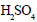solution is nearly
Solution:
QUESTION: 6

0.7 g of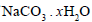is dissolved in 10 mL of water, 20mL of which required 19.8 mL of 0.1 N HCl. The value of x is

Solution:
QUESTION: 7
The energy of the second Bohr orbit of the hydrogen atom is –3.41 eV. The energy of the third Bohr orbit of the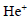ion will be
Solution:
QUESTION: 8

The energy of an electron in the first Bohr orbit of H-atom is –13.6 eV. The possible energy values(s)
of the excited state(s) for electrons in Bohr orbits of hydrogen is (are)

Solution:
QUESTION: 9
The number of nodal planes in a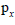orbital is
Solution:
QUESTION: 10
The ratio of time periods in first and second orbits of hydrogen atom is
Solution:
QUESTION: 11
The sub-shell that comes after f-sub-shell is called g-sub-shell. The number of g-sub orbitals in g-sub shell and the total number of orbitals in the principal orbital respectively are
Solution:
QUESTION: 12
The e/m for positive rays in comparison to cathod rays is
Solution:
QUESTION: 13

If n and l are respectively the principal and azimuthal quantum numbers, then the expression for calculating total number of electrons in any energy level is

Solution:

If n and l are principal and azimuthal quantum numbers respectively, then the expression for calculating the total number of electrons in any energy level is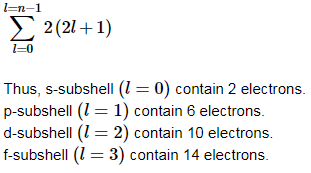QUESTION: 14
The law of triads is applicable to
Solution:
QUESTION: 15
Mendellev’s periodic table is upset by the fact that
Solution:
QUESTION: 16
Name the two elements that are liquid at ordinary temperature
Solution:
QUESTION: 17
Traid-I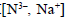Traid-II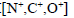Choose the species of lowers IP from traid-I and the species of highest IP from traid-II respectively
Solution:
QUESTION: 18

Arrange the elements with the following electronic configurations in increasing order of electron affinity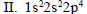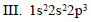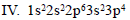Solution:
QUESTION: 19
The IUPAC name of the compound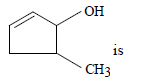Solution:
QUESTION: 20
The IUPAC name of the compound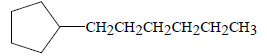Solution:
QUESTION: 21
The IUPAC name of the compound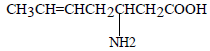is
Solution:
QUESTION: 22
Which of the following shows tautomerism ?
Solution:
QUESTION: 23
Which of the following is the correct order of stability of the following four distinct conformations of nbutane?
Solution:
QUESTION: 24

Which among the following is likely to show geometrical isomerism?

Solution:
QUESTION: 25
Which one of the following is the stablest structure of cyclohexatriene ?
Solution:
QUESTION: 26
meso-Tartaric acid is optically inactive due to the presence of
Solution:
QUESTION: 27
The absolute cofiguration of the following compound is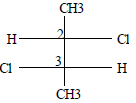Solution:
QUESTION: 28
The strucutre of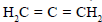is
Solution:
QUESTION: 29
The most stable carbonation among the following is
Solution:
QUESTION: 30

Toluene has

Solution: